Custom Search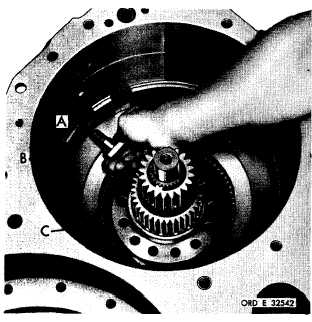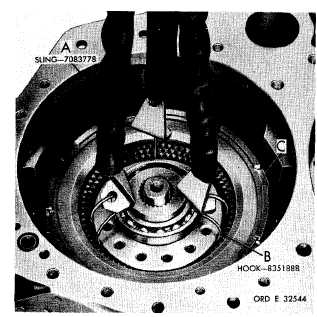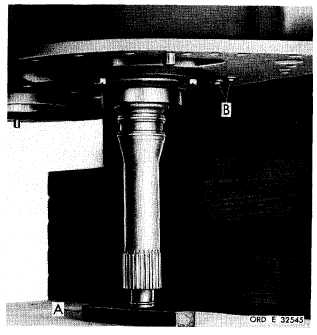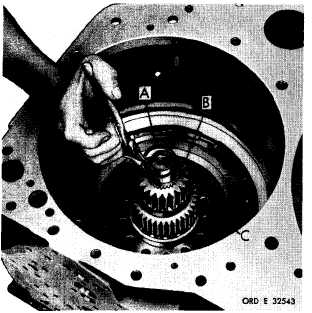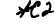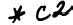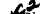*C2 *C2 P A R   75,   STEPS   5 9 - 6 2 D I S A S S E M B L Y C H A P   5,   SEC   I I Figure   123   (Step   59) R e m o v e     i n t e r m e d i a t e - r a n g e     c 1 u t c h     a n c h or b o l t    ( A ) .    R e m o v e    c l u t c h    a n c h o r    ( B )    a n d    o il c o l l e c t o r    ( C ). F i g u r e    1 2 5    ( S t e p    6 1) Using   sling   (A)   and   hooks   (B),   remove   high- range   clutch   assembly   (C). Figure   124   (Step   60) Remove   snap   ring   (A)   retaining   low-range   sun gear   (B).   Remove   low-   (B)   and   intermediate- (C)   range   sun   gears. Figure   126   (Step   62) Block   up   the   turbine   shaft   with   a   wooden   block (A).   Using   a   7/16-inch   wrench,   remove   two self-locking   bolts   (B)   and   flat   washers. 9 9